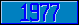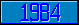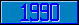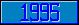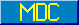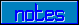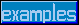## \$%ARCSIN

### M[UMPS] by Example

```
2-Jul–96, 6:54:35

Routine Save for Trigonometry Library function \$%ARCSIN^MATH

;

; Unless otherwise noted, the code below

; was approved in document X11/95–12

;

; If corrections have been applied,

; first the original line appears,

; with three semicolons at the beginning of the line.

;

; Then the source of the correction is acknowledged,

; then the corrected line appears, followed by a

; line containing three semicolons.

;

ARCSIN(X) ;

; Winfried Gerum (8 June 1995)

;  Comment: This version of the function is

;           optimized for speed, not for precision.

;           The 'precision' parameter is not supported,

;           and the precision is at best 2 in 10**–8.

;

New A,N,R,SIGN,XX

If X<–1 Set \$Ecode=",M28,"

If X>1 Set \$Ecode=",M28,"

Set SIGN=1 Set:X<0 X=-X,SIGN=–1

Set A(0)=1.5707963050,A(1)=–0.2145988016,A(2)=0.0889789874

Set A(3)=–0.0501743046,A(4)=0.0308918810,A(5)=–0.0170881256

Set A(6)=0.0066700901,A(7)=–0.0012624911

Set R=A(0),XX=1 For N=1:1:7 Set XX=XX*X,R=A(N)*XX+R

;

;;; Set R=\$%SQRT^MATH(1-X)*R

; Winfried Gerum (8 June 1995)

Set R=\$%SQRT^MATH(1-X,11)*R

;;;

;

Set R=\$%PI^MATH()/2-R

Quit R*SIGN

;===

;

;

ARCSIN(X,PREC) ;

New L,LIM,K,SIGS,VALUE

Set PREC=\$Get(PREC,11)

;

;;; If \$Translate(X,"-")=1 Do  Quit

; Winfried Gerum (8 June 1995)

; Eli Reidler (28 June 1996)

If \$Translate(X,"-")=1 Do  Quit VALUE

. ;;;

. ;

. Set VALUE=\$%PI^MATH()/2*X

. Quit

;

;;; If X>0.99999 Do  Quit

; Winfried Gerum (8 June 1995)

; Eli Reidler (28 June 1996)

If X>0.99999 Do  Quit VALUE

. ;;;

. ;

. Set SIGS=\$Select(X<0:–1,1:1)

. Set VALUE=1/(1/X/X–1)

. ;

. ;;; Set X=\$%SQRT^MATH(VALUE)

. ; Winfried Gerum (8 June 1995)

. Set X=\$%SQRT^MATH(VALUE,PREC)

. ;;;

. ;

. ;;; Set VALUE=\$%ARCTAN^MATH(X,10)*SIGS

. ; Winfried Gerum (8 June 1995)

. Set VALUE=\$%ARCTAN^MATH(X,PREC)*SIGS

. ;;;

. ;

. Quit

Set (VALUE,L)=X

Set LIM=\$Select((PREC+3)'>11:PREC+3,1:11),@("LIM=1E-"_LIM)

For K=3:2 Do  Quit:(\$Translate(L,"-")<LIM)

. Set L=L*X*X*(K–2)/(K–1)*(K–2)/K,VALUE=VALUE+L

. Quit

Quit VALUE

;===

;

;

;

```